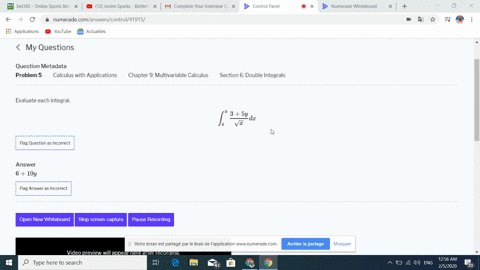🎉 The Study-to-Win Winning Ticket number has been announced! Go to your Tickets dashboard to see if you won! 🎉View Winning Ticket## Discussion

You must be signed in to discuss.

## Video Transcript

Okay, so we have another integral here. Your girl From three to six of dysfunction. X square it X squared plus three. Why d X So why were just thinking is being fixed for announce. Our final answer will be a function of why and now we see we have X squared plus three. Why? And if I differentiate this I'm going to get our with respect to X I'm going to get a constant multiple effects. So that's our hand to let you be X plus square is to apply. Differentiating with respect to X gives us two x the ex wise constants and just goes away. So x T x is one half to you now I can make her see institutions. So in X is equal to three we have you is nine plus three. Why exit six New is thirty six plus three. Why? And then this is just three U that we have this factor of one half when we substitute in for to you. Okay, so anti derivative of squared of you. You know, the one half is just, uh okay. You to the three house divided by three house and then evaluated from nine plus three. Why two thirty six plus three? Why? And so we have one half dividing by three halves that's going to be one third. And then we'LL evaluate you at the upper limits and then subtract evaluating at the lower limit and that sort of thing.